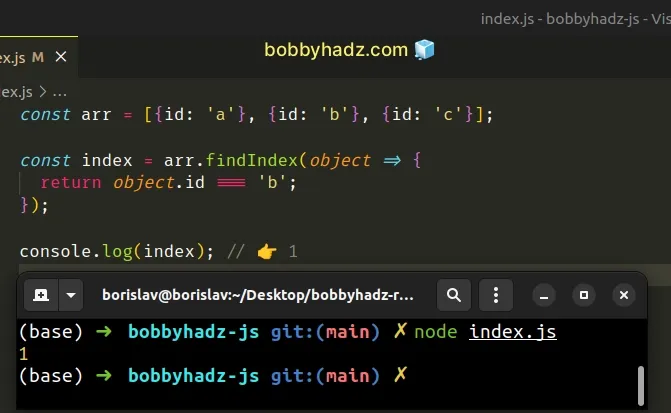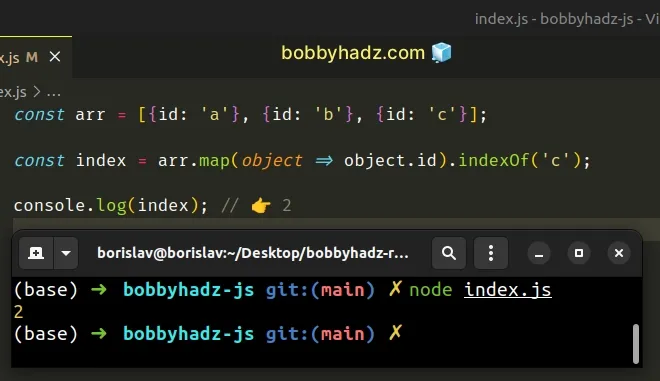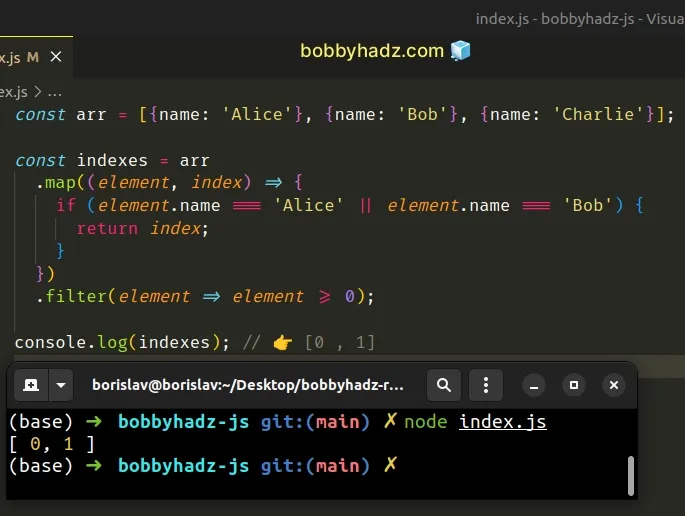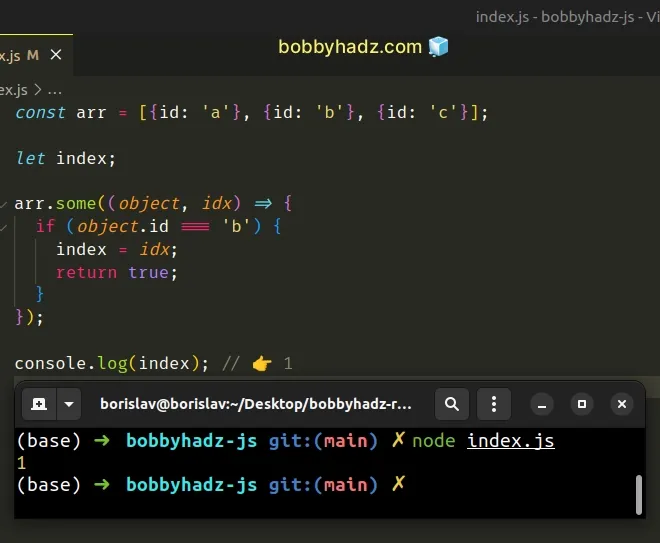# Get the index of an Object in an Array in JavaScriptLast updated: Jan 8, 2023
5 min## #Get the index of an Object in an Array in JavaScript

To find the index of an object in an array by a specific property:

1. Use the `findIndex()` method to iterate over the array.
2. Check if each object has a property with the specified value.
3. The `findIndex()` method will return the index of the first object that matches the condition.
index.js
```Copied!```const arr = [{id: 'a'}, {id: 'b'}, {id: 'c'}];

const index = arr.findIndex(object => {
return object.id === 'b';
});

console.log(index); // 👉️ 1
``````The function we passed to the Array.findIndex() method gets called with each element (object) in the array until it returns a truthy value or iterates over all array elements.

On each iteration, we check if the object's property is equal to a specific value and return `true` or `false`.

The `findIndex()` method returns the index of the first object that meets the condition.

If the function we passed to the `findIndex()` method never returns a truthy value, the method returns `-1`.

Alternatively, you can use the `Array.map()` method.

## #Get the index of an Object in an Array using Array.map()

This is a three-step process:

1. Use the `map()` method to iterate over the array.
2. Return only the values of the relevant property.
3. Use the `indexOf()` method to get the index of the object in the array.
index.js
```Copied!```const arr = [{id: 'a'}, {id: 'b'}, {id: 'c'}];

const index = arr.map(object => object.id).indexOf('c');

console.log(index); // 👉️ 2
``````We used the Array.map() method to get an array containing the values of the specified property.

index.js
```Copied!```const arr = [{id: 'a'}, {id: 'b'}, {id: 'c'}];

const values = arr.map(object => object.id)
console.log(values) // 👉️ ['a', 'b', 'c']
``````

The last step is to use the Array.indexOf method to get the index of the value in the array.

The `map()` method iterates over all of the array's elements, so the ordering of the elements is preserved.

The order of the elements is the same for both the array of values and the array of objects.

The `indexOf` method returns the index of the first object that meets the condition.

If the `indexOf()` method doesn't find an element with the given value, it returns `-1`, just like the `findIndex()` method.

I've also written a detailed guide on how to filter an array of objects.

## #Get the Indexes of all Objects in an Array that match a condition

To get all indexes of all objects in an array that match a condition:

1. Use the `map()` method to iterate over the array.
2. Check if each object matches the condition and return the matching indexes.
3. Use the `filter()` method to remove all `undefined` values from the array.
index.js
```Copied!```const arr = [{name: 'Alice'}, {name: 'Bob'}, {name: 'Charlie'}];

const indexes = arr
.map((element, index) => {
if (element.name === 'Alice' || element.name === 'Bob') {
return index;
}
})
.filter(element => element >= 0);

console.log(indexes); // 👉️ [0 , 1]
``````The function we passed to the Array.map() method gets called with each element (object) in the array.

If the condition is met, we return the element's index, otherwise, the function implicitly returns `undefined`.

The return value of the `map()` method will contain the indexes of the array elements that meet the condition and an `undefined` value for each element that doesn't.

index.js
```Copied!```const arr = [{name: 'Alice'}, {name: 'Bob'}, {name: 'Charlie'}];

// 👇️ [0, 1, undefined]
console.log(
arr.map((element, index) => {
if (element.name === 'Alice' || element.name === 'Bob') {
return index;
}
}),
);
``````

We used the Array.filter method to remove the `undefined` values from the array.

In the callback function we passed to the `filter()` method, we check if the element is greater than or equal to `0`.

The `filter` method returns a new array that contains the indexes of all elements that meet the condition.

You can also use the `Array.some()` method to get the index of an object in an array.

If you need to update an object's property in an array of objects, follow the instructions in this article.

## #Get the index of an Object in an Array using `Array.some()`

This is a three-step process:

1. Use the `Array.some()` method to iterate over the array.
2. Check if the current object has a property equal to the specified value.
3. If the condition is met, assign the current index to a variable.
index.js
```Copied!```const arr = [{id: 'a'}, {id: 'b'}, {id: 'c'}];

let index;

arr.some((object, idx) => {
if (object.id === 'b') {
index = idx;
return true;
}
});

console.log(index); // 👉️ 1
``````The function we passed to the Array.some() method gets called with each element (object) in the array.

If at least one invocation of the callback function returns a truthy value, the `some()` method returns `true`, otherwise, `false` is returned.

On each iteration, we check if the current object has an `id` property with the specified value.

If the condition is met, we assign the current index to the `index` variable and return `true` to break out of the loop.

You can initialize the `index` variable to a different value, e.g. `-1` if you want the variable to store `-1` in case an object with the specified value isn't found.

index.js
```Copied!```const arr = [{id: 'a'}, {id: 'b'}, {id: 'c'}];

let index = -1;

arr.some((object, idx) => {
if (object.id === 'ASDF') {
index = idx;
return true;
}
});

console.log(index); // 👉️ -1
``````

None of the objects in the array meets the condition, so the `index` variable remains set to `-1`.

Want to learn more about working with an array of objects in JavaScript? Check out these resources:

## #Get the index of an Object in an Array using a `for` loop

This is a three-step process:

1. Use a `for` loop to iterate over the array.
2. Check if the current object has a property with the specified value.
3. If the condition is met, assign the current index to a variable.
index.js
```Copied!```const arr = [{id: 'a'}, {id: 'b'}, {id: 'c'}];

let index;

for (let idx = 0; idx < arr.length; idx++) {
if (arr[idx].id === 'b') {
index = idx;
break;
}
}

console.log(index); // 👉️ 1
``````

We used a `for` loop to iterate over the array of objects.

On each iteration, we check if the current object has an `id` property equal to a specific value.

If the condition is met, we assign the current index to a variable and exit the `for` loop.

The `break` statement is used to end the `for` loop prematurely to avoid iterating needlessly.